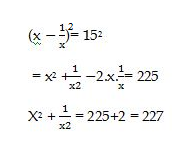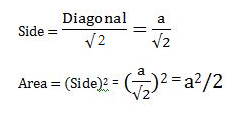# RRB ALP 2018 Practice Test Papers | Arithmetic Questions (Day-49)

Dear Aspirants, Here we have given the Important RRB ALP & Technicians Exam 2018 Practice Test Papers. Candidates those who are preparing for RRB ALP 2018 can practice these Arithmetic Questions to get more confidence to Crack RRB 2018 Examination.

[WpProQuiz 2648]

Click “Start Quiz” to attend these Questions and view Explanation

1. If 1 man or 2 Women or 3 Children can do a piece of work in 2 days, then the same piece of work will be done by 1 man and 1 woman and 1 child
1. 16 days
2. 6/7 days
3. 12/11 days
4. 8 days
1. A man can row a Boat 16 km in 2 hours downstream and returns upstream in 4 hours. The speed of the Stream is
1. 4 kmph
2. 2 kmph
3. 2.5 kmph
4. 3 kmph
1. if p,q,r are in Geometric Progression, then which of the following is true?
1. q = (p+r)/2
2. p2 =qr
3. q = √pr
4. p/r=r/q
1. If (x – 1)/x = 15, then x2 + 1/x2 is
1. 225
2. 222
3. 227
4. 220
1. Find the Value of

tan 5°. tan 44°. tan 46°. tan 85°

1. 1
2. 0
3. 2
4. 3
1. The length of the diagonal of a Square is ‘a’cm. Which of the following represents the area of the square?
1. 2a
2. a2/2
3. a/ √2
4. 4a
1. 9x2 +25 – 30x can be expressed as the Square of
1. −3x – 5
2. 3x + 5
3. 3x2 – 25
4. 3x – 5
1. A 250Meter long train is moving at 10kmph. It will cross a man standing on the platform in
1. 3 min
2. 1 min
3. 1.5 min
4. 2 mins
1. Simplify

600 + 45% of 50 – x = 75% of 800 + √169

1. 8.5
2. 7.5
3. 6.5
4. 5.5
1. In a vessel contains Milk and water in the ratio of 5:3. How much more water to be added so that the milk and water ratio will be 2:3?
1. 3
2. 3.5
3. 4
4. 4.5

1M = 2W = 3C

1M = 3C

1W = 3/2C

1M+1W+1C = 3C + 3/2C+1C = 11/2 C

3C è 2 Days

11/2 è ?

(3×2×2) / 11 = 12/11 days

Downstream speed= 16/2 = 8kmph

Upstream speed=  16/4= 4kmph

Speed of the stream = (x-y)/2 = (8 – 4)/2 = 4/2 = 2 kmph

∴ q/p = r/ q

⇒ q2 = pr

⇒ q = √prtan 5°.tan 44°. tan (90° – 44°). tan (90° – 5°)

= tan 5° ⨯tan 44°⨯ cot 44°⨯ cot 5°

= tan 5°⨯cot 5° ⨯ cot 44° ⨯ tan 44° = 1 [tanθ.cotθ = 1]9x2 – 30x +25

=9x2 – 15x – 15x + 25

=3x (3x – 5) – 5(3x – 5)

= (3x – 5)2

Speed = 10 × 5/18 = 50/18

Time = Distance / speed

= (250 ×18)/50 = 90 sec

= 1.5mins

600+22.5 – x = 600+13

622.5 – x= 613

X = 622.5 – 613

X= 9.5

5/(3+x) = 2/3

15= 6+2x

2x= 9

X= 4.5

RRB ALP 2018 – “All in One” Study Materials and Practice Sets